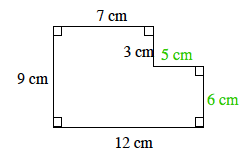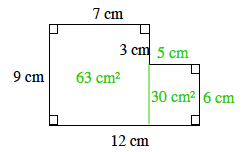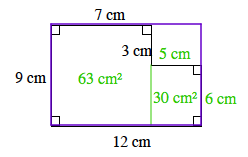### Home > CC1 > Chapter 7 > Lesson 7.2.2 > Problem7-54

7-54.

Find the area of the shape below in at least two ways. Homework Help ✎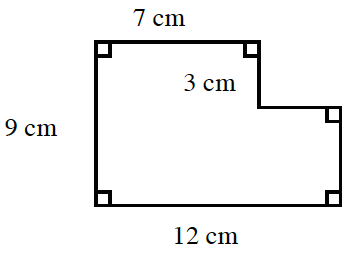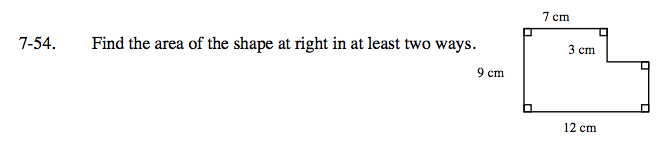Label each remaining side using centimeters.

Our first method of finding the area is to divide the shape into smaller rectangles and label their areas.

(9)(7) + (5)(6) = 63 + 30 = 93 square centimeters

Another method for finding the area is to subtract the area of the rectangle in the upper-right corner from the area of the purple rectangle. Show that this method gives the same answer as Method 1.